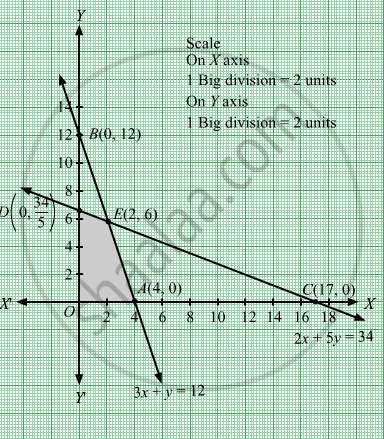# Maximize Z = 10x + 6y Subject to 3 X + Y ≤ 12 2 X + 5 Y ≤ 34 X , Y ≥ 0 - Mathematics

Sum

Maximize Z = 10x + 6y
Subject to

$3x + y \leq 12$
$2x + 5y \leq 34$
$x, y \geq 0$

#### Solution

First, we will convert the given inequations into equations, we obtain the following equations:
3x y = 12, 2x + 5y = 34, x = 0 and y = 0
Region represented by 3x y ≤ 12:
The line 3x y = 12 meets the coordinate axes at $A\left( 4, 0 \right)$ and  $B\left( 0, 12 \right)$ respectively. By joining these points we obtain the line 3x y = 12.
Clearly (0,0) satisfies the inequation 3x y ≤ 12. So,the region containing the origin represents the solution set of the inequation 3x y ≤ 12 .
Region represented by 2x + 5y  ≤ 34:
The line 2x + 5y = 34 meets the coordinate axes at $C\left( 17, 0 \right)$ and  $D\left( 0, \frac{34}{5} \right)$

respectively. By joining these points we obtain the line 2x + 5y  ≤ 34.
Clearly (0,0) satisfies the inequation 2x + 5y  ≤ 34. So,the region containing the origin represents the solution set of the inequation 2x + 5y  ≤ 34.

Region represented by x ≥ 0 and y ≥ 0:
Since, every point in the first quadrant satisfies these inequations. So, the first quadrant is the region represented by the inequations x ≥ 0 and ≥ 0.

The feasible region determined by the system of constraints, 3x y ≤ 12, 2x + 5y  ≤ 34, x ≥ 0, and y ≥ 0 are as follows.The corner points of the feasible region are O(0, 0), $A\left( 4, 0 \right)$, $E\left( 2, 6 \right)$ and $D\left( 0, \frac{34}{5} \right)$ .

The values of Z at these corner points are as follows:

 Corner point Z = 10x + 6y O(0, 0) 10 × 0 + 6 × 0 = 0 $A\left( 4, 0 \right)$ 10× 4 + 6 × 0 = 40 $E\left( 2, 6 \right)$ 10 × 2 + 6 × 6 = 56 $D\left( 0, \frac{34}{5} \right)$ 10 × 0 + 6 × $\frac{34}{5}$ = $\frac{204}{3}$

We see that the maximum value of the objective function Z is 56 which is at $E\left( 2, 6 \right)$ that means at = 2 and y = 6.
Thus, the optimal value of Z is 56.

Concept: Graphical Method of Solving Linear Programming Problems
Is there an error in this question or solution?

#### APPEARS IN

RD Sharma Class 12 Maths
Chapter 30 Linear programming
Exercise 30.2 | Q 7 | Page 32